• 面向对象基础练习 类的简单实用 案例: 学生类Student 类属性 count 记录创建学生个数 ... 类属性 country 学生国家 ... 实例属性含有名字，年龄，性别... 创建一个私有方法study打印xx(xx为姓名): 在学习 创建...
 面向对象基础练习
类的简单实用

案例:
学生类Student
类属性 count 记录创建学生个数
类属性 country 学生国家
实例属性含有名字，年龄，性别,钱（默认为0，私有属性）,国家
国家默认与类属性国家相同
创建对象属性country的get和set方法
创建对象私有属性钱的get和set方法
创建一个私有方法study打印xx(xx为姓名): 在学习
创建一个上课方法调用私有方法 创建answer方法 打印 xx:在回答问题
创建一个学生(张三,20,男) 调用所有的方法
再创建一个学生(Tom,19,男) 设置学生的国籍为美国 设置金钱为10000 调用所有方法查看
再创建一个学生(吴敏,18,女） 调用所有方法

代码如下:
# _*_ coding: utf-8 _*_
# Date: 2019/8/11 0011 12:29
# Author: Mijiu
# Version: 1.0

class Student:
count = 0   # 类属性 记录班里一共多少个学生

def __init__(self, name, sex, age,):      # 初始化,构造函数
self.name = name
self.age = age
self.sex = sex
self.__money = 0
print(f"欢迎新同学{self.name},{self.sex},{self.age}")
Student.count += 1    # 每次被实例化一次班里学生+1

country = "中国"

def get_country(self):         # 获取国家
return self.country

def set_country(self,country):    # 修改类属性
self.country = country
def get_money(self):
print(f"{self.name} 余额:{self.__money}")
return self.__money

def set_money(self,money):        # 修改类属性money
self.__money = money

def __study(self):             # 私有方法  在外部无法直接调用
print(f"{self.name}:在学习")

def answer(self):
print(f"{self.name}:在回答问题")

def class_(self):             # 公有方法调用调用私有方法
self.__study()

def main():

zs = Student("张三","男",20) # 张三,20,男
print(f"国家  {zs.get_country()}")
zs.get_money()
zs.class_()
zs.answer()

Tom = Student("Tom","男",19)           #Tom, 19, 男
Tom.set_money(10000)            # 余额修改为10000
Tom.set_country("美国")         # 改变国籍为美国
print(f"国家   {Tom.get_country()}")
Tom.get_money()
Tom.class_()
Tom.answer()

zss = Student("吴敏", "女", 18)  # 吴敏,18,女
print(f"国家  {zss.get_country()}")
zss.get_money()
zss.class_()
zss.answer()
print (f"班级学生总数为:{Student.num}位同学")
main()




展开全文• 使用python创建学生管理系统，供大家参考，具体内容如下 创建学生管理系统，可谓是学习编程最基础的小步。 主要是分为以下几思路： 接下来直接上源码 #!/usr/bin/python3 # coding=utf-8 # 导入包 import ...
• ## 使用python创建学生管理系统

千次阅读 多人点赞 2019-11-17 11:36:05
使用python创建学生管理系统 创建学生管理系统，可谓是学习编程最基础的小步。 主要是分为以下几思路： 接下来直接上源码 #!/usr/bin/python3 # coding=utf-8 # 导入包 import random # 全局变量 # 函数 # #...
使用python创建学生管理系统
创建学生管理系统，可谓是学习编程最基础的一小步。
主要是分为以下几个思路：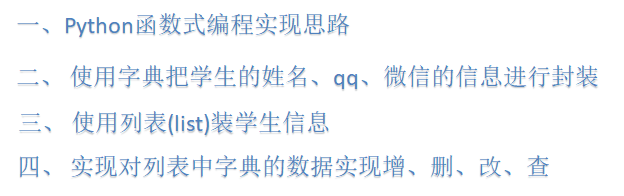接下来直接上源码
#!/usr/bin/python3
# coding=utf-8
# 导入包
import random
# 全局变量
# 函数
# 类
# 调用函数或者类
# 装所以数据的列表
class StudentSys(object):
def __init__(self):
# 实例属性
self.names = []
self.infos = "1:增加|2:删除|3:修改|4:查找|5:显示|6:退出系统"

# 打印提示信息(菜单)
def print_menu(self):
"打印提示菜单信息"
print("=" * 25)
print("\t~学生管理系统版本~")
print("\t1:添加学生信息")
print("\t2:删除学生信息")
print("\t3:修改学生信息")
print("\t4:查找学生信息")
print("\t5:显示学生信息")
print("\t6:退出学生信息系统")
print("=" * 25)

def add_info(self):
"添加信息"
self.student = {}
name = input("请添加新同学的姓名:")
phone = input("请添加新同学的手机号:")
wechat = input("请输入新同学的微信号:")
self.student["name"] = name
self.student["phone"] = phone
self.student["wechat"] = wechat

# 把学生信息(字典里面)添加到列表
self.names.append(self.student)
self.show_info()
self.save_info()

def delete_info(self):
'删除信息'
# 根据下标删除和内容删除和末尾删除
del_name = input("请输入删除的学生的姓名:")
for name in self.names:
# name:{"name":"曹操"....}
if del_name == name.get("name"):
self.names.remove(name)

self.show_info()
self.save_info()

def modify_info(self):
"""修改信息"""
# 根据下标修改index是列表的方法
find_name = input("请输入您需要修改学生的姓名: ")
flag = 0  # 0,没有找到,1找到了
for name in self.names:

if find_name == name["name"]:
new_name = input("请输入新的名字: ")
name["name"] = new_name
flag = 1
break

if flag == 0:
print("该名学生%s不存在" % find_name)

else:
self.show_info()
self.save_info()

def find_info(self):
'''查找信息'''
find_name = input("请输入你要查找的姓名:")
flag = 0  # 0,没有找到,1找到了
for name in self.names:
for value in name.values():
if find_name == value:
flag = 1
print("找到了:{}".format(find_name))
break

if flag == 0:
print("没有找到:{}".format(find_name))

def show_info(self):
"表格方式显示所以信息"
print("\n")
print("当前学生信息")
print("~" * 50)
print("\t姓名\t\t\t电话\t\t\t微信\t")
for name in self.names:
msg = "\t" + name.get("name") + "\t\t" + name.get("phone") + "\t\t" + name.get("wechat") + "\t\t"
print("~" * 50)
print(msg)
print("~" * 50)
print("\n")

# 程序的主要逻辑和程序入口
def start(self):
self.print_menu()
# 加载文件中保存的信息,加载到内存中
self.load_info()
while True:
print("\n\n")
print("操作指令")
print("~" * 50)
print(self.infos)
print("~" * 50)
number = input("请按照上面的提示输入相应指令:")

# 判断是否输入是纯的数字
if number.isdigit():
number = int(number)
if number == 1:
# 添加信息
self.add_info()
elif number == 2:
# 删除信息
self.delete_info()
elif number == 3:  # 修改
# 修改信息
self.modify_info()
elif number == 4:  # 查找
# 查找信息
self.find_info()
elif number == 5:
# 显示信息
self.show_info()
elif number == 6:
break

else:
print("请输入正确的编号!")

# 运行的时候,读取保存在文件的信息,并且赋值给names,第一次读文件,文件不存在,"r"会报错,"a+"
def load_info(self):
f = open("students.txt", "a+")
f.seek(0, 0)
content = f.read()
# print("content==",content)
if len(content) > 0:
self.names = eval(content)

# 每次删除或者修改或者增加都重新保存数据,覆盖保存w
def save_info(self):
f = open("students.txt", "w")
f.write(str(self.names))
f.close()

s = StudentSys()
s.start()

实现后的功能如下：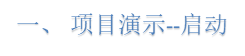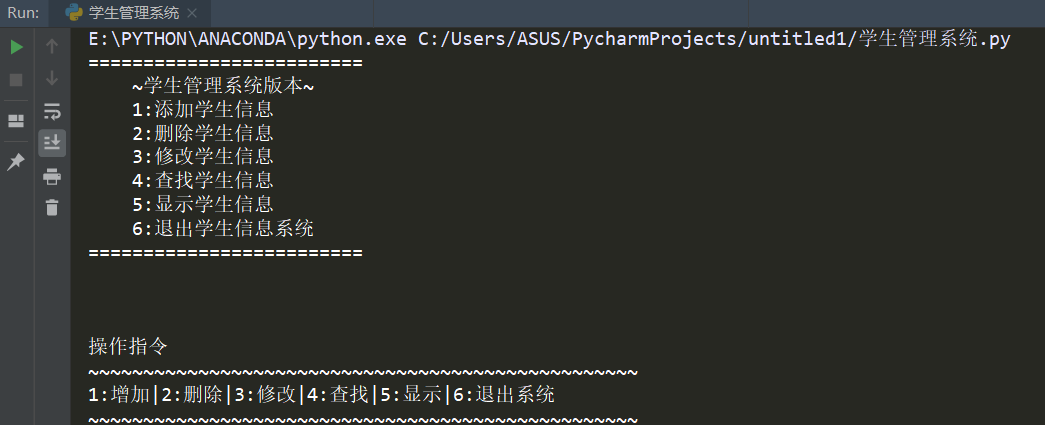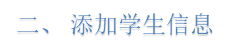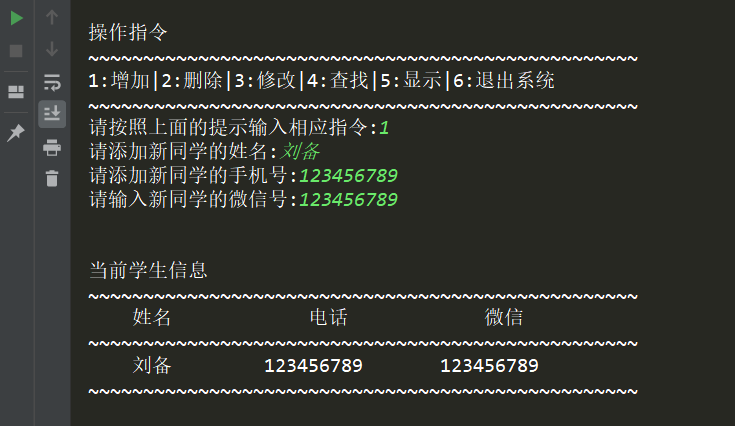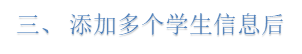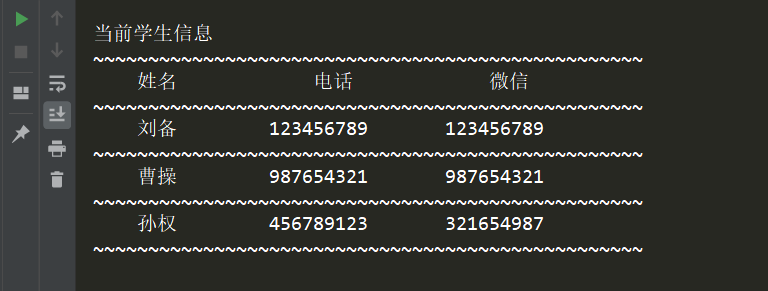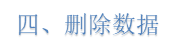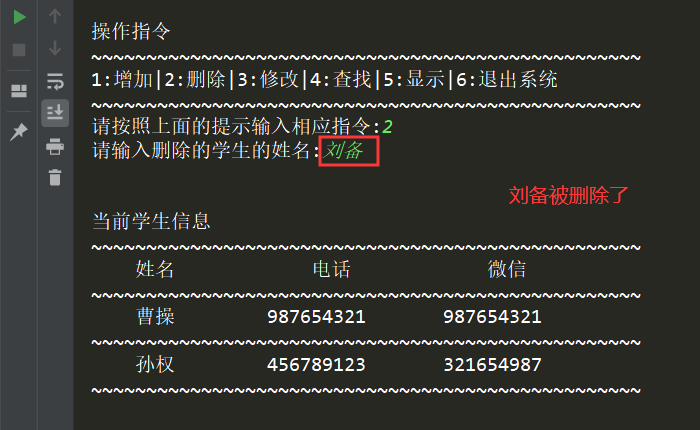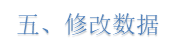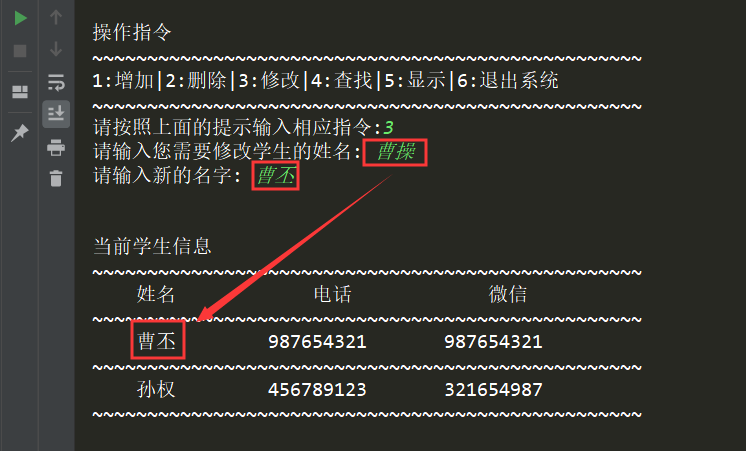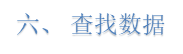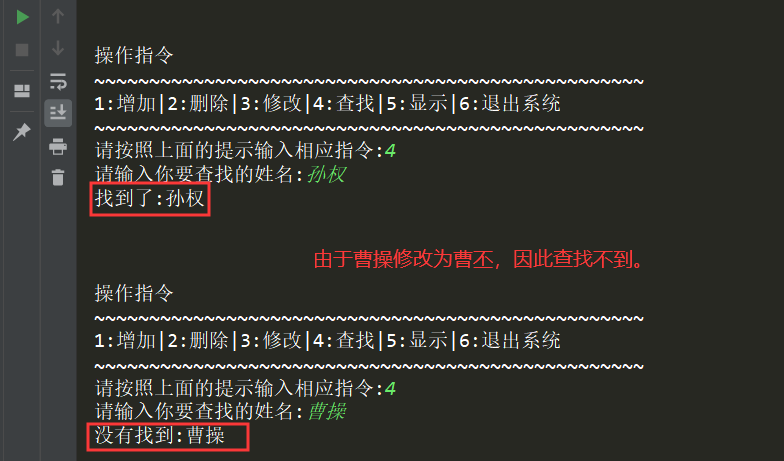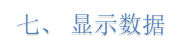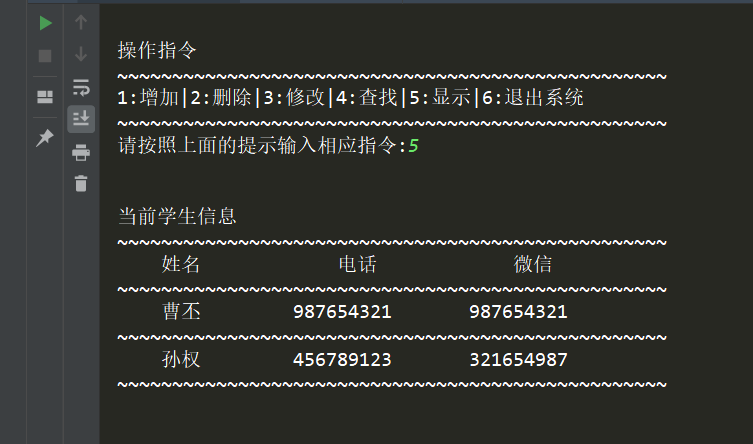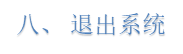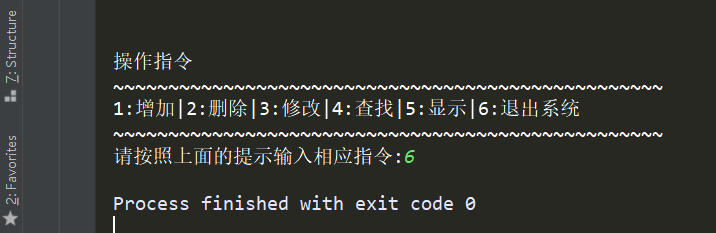基本思路就是这样，希望对你有所帮助。


展开全文• 要求编写学生类，班级类，并在电脑运行生成表单，输入一个数字，得到对应的结果。 输出样式 代码如下 学生类 class Student: def __init__(self,sno,name,english,math): self.sno=sno self.name=name self.__...
• python类的创建 在这里插入代码片 """ 定义学生类 """ class Student(): name = None age = 18 coures = 'python' #定义方法 def doHomeWork(self): ...#实例化一个学生 yueyue...
python类的创建
在这里插入代码片
"""
定义学生类
"""
class Student():
name = None
age = 18
coures = 'python'

#定义方法
def doHomeWork(self):
print('I 在做作业')
return  None

#实例化一个学生
yueyue = Student()
print(yueyue.name)
print(yueyue.age)
#使用方法
yueyue.doHomeWork();
#检查对象的成员
yueyue.__dict__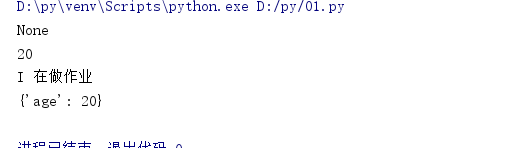展开全文• 要求编写学生类，班级类，并在电脑运行生成表单，输入一个数字，得到对应的结果。 输出样式 代码如下 学生类 class Student: def __init__(self,sno,name,english,math): self.sno=sno self.name=name self.__...
python学生成绩管理系统创建
要求编写学生类，班级类，并在电脑运行生成表单，输入一个数字，得到对应的结果。
输出样式代码如下
学生类
class Student:
def __init__(self,sno,name,english,math):
self.sno=sno
self.name=name
self.__english=english
self.__math=math

@property
def english(self):
return self.__english

@english.setter
def english(self,english):
self.__english=english if 0<=english<=100 else 0

@property
def math(self):
return self.__math

@math.setter
def math(self,math):
self.__math=math if 0<=math<=100 else 0

def get_average(self):
return (self.english+self.math)/2

def __str__(self):
s=str.format('{0:>4d}{1:　>4s}{2:>8d}{3:>8d}',self.sno,self.name,self.english,self.math)
return s

@staticmethod
def get_header():
return  '{0:>2s}{1:　>4s}{2:\u3000>4s}{3:　>4s}'.format('学号','姓名','英语','数学')

def __eq__(self,other):
return self.sno==other.sno

def __gt__(self,other):
return self.sno>other.sno

测试
#测试
if __name__=='__main__':
s1=Student(1,'王大海',100, 65)
s2=Student(2,'李三',38,45)
s3=Student(3,'李四',88,45)
print(s1>s2)
students=[s2,s1,s3]
students.sort()
print(students)
print(Student.get_header())
for s in students:
print(s)

结果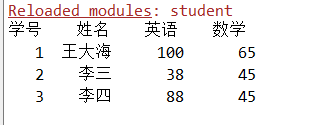班级类
from student import Student

class BanJi:
def __init__(self,name):
self.name=name
self.students=[]
self.load_data()

def load_data(self):
with open('data.txt','r')as f:
for line in f:
d=line.split()
self.students.append(Student(int(d),d,int(d),int(d)))

def show_student(self):
print(Student.get_header())
for s in self.students:
print(s)

def add_student(self,student):
if student in self.students:
return '此学生已存在'
else:
self.students.append(student)
return'添加成功'

def __index_student(self,sno):
s=Student(sno,'',0,0)
if s not in self.students:
return None
else:
return self.students.index(s)

def find_student(self,sno):
i = self.__index_student(sno)
if i is None:
return None
else:
return self.students[i]

def delete_student(self,sno):
i = self.__index_student(sno)
if i is None:
r = '没有学号为{0: d}'.format(sno)
else:
del self.students[i]
r = '删除成功！'
return r

def update_student(self,sno):
s = self.find_student(sno)
if s is None:
return '没有学号为{0：d}的学生！'.format(sno)
else:
print(s)
print('请输入新的值，直接回车不修改！')
name_str = input('姓名：')
english_str = input('英语：')
math_str = input('数学：')
s.name = name_str if len(name_str)>0 else s.name
s.name = int(english_str) if len(english_str)>0 else s.english
s.name = int(math_str) if len(math_str)>0 else s.math
return '修改成功！'

def save_data(self):
with open('data.txt','w',encoding = 'GBK') as f:
for s in self.students:
r = str.format('{0:d} {1:s} {2:d} {3:d}\n',s.sno,s.name,s.english,s.math)
f.write(r)

def sort_by_english(self,reverse = False):
self.students.sort(key=lambda x: x.english,reverse = reverse)

学生管理系统
import os
from student import Student
from banji import BanJi

menu = """

*********************

学生成绩管理系统
1.浏览学生成绩
2.按学号查找学生
3.按英语成绩排序
4.添加学生
5.删除学生
6.修改学生信息
9.保存
0.退出

*********************

"""
tip = '输入（0-9）选择操作：'

choice = ''

b = BanJi('1802')

os.system('cls')
print(menu)

while True:
choice = input(tip)
os.system('cls')
print(menu)
if choice == '0':
print('数据以保存！')
break
elif choice == '1':
b.show_student()
elif choice == '2':
sno = int(input('请输入您需要查询的学生的学号：'))
s = b.find_student(sno)
if s is None:
print('无此学号的学生！')
else:
print(s)
elif choice == '3':
b.sort_by_english()
b.show_student()
elif choice == '4':
print('请输入学生信息：')
sno = int(input('学号：'))
name = input('姓名：')
english = int(input('英语：'))
math = int(input('数学：'))
student = Student(sno,name,english,math)
r = b.add_student(student)
print(r)
elif choice == '5':
sno = int(input('请输入要删除的学生的学号：'))
r = b.delete_student(sno)
print(r)

elif choice == '6':
sno = int(input('请输入要修改的学生的学号：'))
r = b.update_student(sno)
print(r)

elif choice == '9':
b.save_data()
print('保存成功！')
else:
print('输入错误！')

用spyder或者cmd运行都可以。


展开全文• 这里写自定义目录标题欢迎使用Markdown编辑器新的改变功能快捷键合理的创建标题，有助于目录的生成如何改变文本的样式插入链接与图片如何插入一段漂亮的代码片生成一个适合你的列表创建一个表格设定内容居中、居左、...
• 要想具体化，就需要创建一个类的实例，称的实例对象。实例对象中拥有属性和方法，它可以表示任何事物的属性和动作，实例对象就是一个大的集合体。如果想要表示最具体的个体，就需要将实例对象实例化，称实例化对象...
• Python课程设计】学生成绩管理系统1、需求分析2、功能设计与分析1、使用数据库对数据进行存取（1）使用PyMySQL模块操作数据库对数据进行存取（2）创建数据库school，创建数据表student_sore、teacher_login（3）...
• 创建一个学生信息，然后从终端依次输入学生的信息。最后打印出来。   有不对的地方请大家予以指正，非常感谢！！！   #!/usr/bin/env python #Crate A Student Manage System #建立一个学生信息数组 ...
• 我们创建一个简单的学生类----它不表示特定的学生，它可以表示任何学生。对于学生，我们知道学生 拥有一个学号，这是没有重复的，学生有名字、年龄；我们还知道学生可以有学习、运动这些行为。 根据以上描述，我们...
• Python中，定义是通过class关键字，例如我们定义一个存储学生信息的： class Student(object): pass class后面紧接着是类名，即Student，类名通常是大写开头的单词，紧接着是(object)，表示该是从哪个继承...
• 创建一个类方法记录车的品牌mark、颜色color、价格price、速度speed等特征， 并实现增加车辆信息、显示车辆全部信息的功能。 创建一个类方法记录车的品牌mark、颜色color、价格price、速度speed等特征， 并实现增加...
• 如动物可以是一个类，而狗可以是这个一个实例， 人可以是一个类，而学生可以是这个一个实例 2.创建类与实例： 创建 ： class 类名(父类或基类，一般默认为object) ： 属性 方法 实例的创建： ...
• 一. 创建类 1. 的定义 class是用来描述具有相同属性和方法的...# 定义一个类，用来描述学习Python学生 # Pythonstuednt表示的实例 class Pythonstudent (): # 用None表示暂时不确定 name = None age = 20
• 为了统计学生人数，可以给Student增加一个属性，每创建一个实例，该属性自动增加： class Student(object): count = 0 def __init__(self, name): self.name = name Student.count+=1 # 测试: if Student....
• 学生类2. 班级类3.课程类4. 老师类 尝试定义类的必要属性和函数，它们有以下关系: 一名学生属于一个班级，可以注册不同的课程 一门课程包含一个注册学生名单和一个老师，每个学生都有这个课程...有问必答 microsoft
• ## python中的类及self详解

万次阅读 多人点赞 2018-04-04 11:04:37
我们知道，面向对象最重要的概念就是（class）和实例（instance），是抽象的模板，比如学生这个抽象的事物，可以用一个Student来表示。而实例是根据类创建出来的一个个具体的“对象”，每一个对象都从中继承...
• Python中self用法详解 ...而实例是根据类创建出来的一个个具体的“对象”，每一个对象都从中继承有相同的方法，但各自的数据可能不同。 (Object)表示该从哪个继承下来的，Object是所有都会继承的 由...self
• 1、创建4个.py模块，在一个包内，模块命名分别为main.py，ui.py，model.py，bll.py。 2、（1）实现学生管理系统在控制台的输出显示界面如图1所示。 （2）在model.py模块中建立学生类，其中包含的私有属性有name，...编程语言
• 我们知道，面向对象最重要的概念就是（class）和实例（instance），是抽象的模板，比如学生这个抽象的事物，可以用一个Student来表示。而实例是根据类创建出来的一个个具体的“对象”，每一个对象都从中继承...
• # 第一阶段 class Student(object): def __init__(self,name,age,sex): self.name = name self.age = age ...# Student，相当于一个模板，只要给顶它name,age,sex就能创建出来一个学生对象 p
• 为了统计学生人数，可以给Student增加一个属性，每创建一个实例，该属性自动增加： class Student(object): count = 0 def __init__(self, name): self.name = name Student.count+=1
• class Student() :# 定义一个学生类 这样就算定义了一个类 而 通过class创建的 类 类型都是 type 也就是类 一个类里面的 物种 各有各的特点，那么用来记录这种特点的方法就叫 属性 class S...
• Python的定义和理解前言一、的...而实例是根据类创建出来的一个个具体的“对象”，每一个对象都从中继承有相同的方法，但各自的数据可能不同。 一、的定义 python中的是用来描述具有相同的属性和方法的编程语言 面向对象编程 class
• 面向对象的重要概念就是类（Class）和实例（Instance），必须牢记类是抽象的模板，比如学生类Student，而实例是根据类创建出来的一个个具体的对象，每个对象都拥有相同的方法，单各自的数据可能不同。 　以Student...
• 我们知道，面向对象最重要的概念就是（class）和实例（instance），是抽象的模板，比如学生这个抽象的事物，可以用一个Student来表示。而实例是根据类创建出来的一个个具体的“对象”，每一个对象都从中继承...
• 背景：创建一个简单的学生管理系统，熟悉增删改查操作 一：创建一个Django项目(http://www.cnblogs.com/wupeiqi/articles/6216618.html) 1：创建实体 from django.db import models # Create your ......

# python创建一个学生类python 订阅